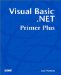# Short-Circuiting Operators

Visual Basic .NET provides two new operators: AndAlso and OrElse . The following sections explain how to use them in your programs.

#### The AndAlso Operator

To appreciate what the new AndAlso operator brings to the table, you have to understand what Visual Basic .NET does with complex expressions in an If statement. Consider the following If statement:

` If Number > 65 And OddEven(Number) = 1 Then `

In this statement, Visual Basic .NET must evaluate two relational comparisons. The first comparison tests whether Number is greater than 65. The second comparison tests whether the function call to OddEven(Number) returns the value 1 . Visual Basic .NET evaluates both comparisons, even if the first test is logic False .

To observe this processing sequence, you can replace the code from the btnTest object's Click() event with the following code:

` Dim Number As Integer  Number = CInt(txtNumber.Text) If Number > 65 And OddEven(Number) > 0 Then  MessageBox.Show("Both true") Else  MessageBox.Show("At least one is not true") End If `

Notice that both comparison expressions use the greater-than relational operator, so their precedence levels are the same. This means the two comparison expressions are evaluated from left to right (that is, Number is evaluated first). You should set a breakpoint on the Mod statement in the OddEven() function. Then compile and run the program, entering the value 1 in the txtNumber text box. The program pauses at the breakpoint in the OddEven() function.

Because Visual Basic .NET stops at the breakpoint, you know that Visual Basic .NET executed the call to OddEven() , even though the first expression evaluated to logic False . This is inefficient because if the first expression is logic False , the truth table for logic And (refer to Table 10.3 in Chapter 10) tells you that regardless of the outcome of the second expression, there is no way the Then statement block can be executed. The logical And operator requires that both expressions be logic True in order for the Then statement block to execute. However, after the first comparison on Number , a logic False condition exists. Why even bother making the call to OddEven() ? Visual Basic .NET is going to execute the Else statement block regardless of what OddEven() returns. Indeed, it is wasteful to make the call to OddEven() .

Now change the If statement to this:

` If Number > 65 AndAlso OddEven(Number) > 0 Then `

The only change in the statement is the use of the AndAlso operator. Now you should keep the breakpoint in the OddEven() function and recompile the program. If you now run the program, using the value 1 for txtNumber , what happens?

The program still displays the message for the Else statement block, but the breakpoint is never reached. This means that because the first relational test on Number returned logic False , Visual Basic .NET said, "The heck with the call to OddEven() ; what's the point?" In other words, the AndAlso operator allows Visual Basic .NET to short-circuit the call to OddEven() if its relational test cannot alter the outcome of the complex expression. This allows you to avoid an unnecessary call to OddEven() .

Programmer's TipYou can gain efficiency with the AndAlso operator only when the first expression evaluates to logic False . Therefore, if you plan to use the AndAlso operator, you should place the expression that is most likely to be False on the left side of the AndAlso operator.

#### The OrElse Operator

As you might expect, there is a short-circuit operator for the logic Or relational operator: the OrElse operator. To understand how it works, you can simply change the AndAlso operator in the If statement of the btnTest object's Click() event to OrElse :

` If Number > 65 OrElse OddEven(Number) > 0 Then `

The intention is different now, but a little thought will show what's going on. What you want to do now is execute the Then statement block if Number is greater than 65 or if OddEven() returns a value greater than 1. From the Or truth table in Chapter 10 (that is, Table 10.4), you know that if either relational test is logic True , Visual Basic .NET should execute the Then statement block. Another way of stating this is "If Number is greater than 65, why bother calling the OddEven() function?" When the first relational test is True , OrElse allows you to short-circuit the call to OddEven() .

You should now compile the program, but keep the breakpoint in the OddEven() function. Run the program, and enter 66 in the txtNumber text box. The program executes the Then statement block without calling the OddEven() function. (The messages that are displayed are messed up because you changed the logic from a test based on an And comparison to one using an Or , but you should get the idea.) Now try entering a value less than 65 and explain what happens.

Programmer's TipYou can gain efficiency with the OrElse operator only when the first expression evaluates to logic True . Therefore, if you plan to use the OrElse operator, you should place the expression that is most likely to be True on the left side of the OrElse operator.Visual Basic .NET Primer Plus
ISBN: 0672324857
EAN: 2147483647
Year: 2003
Pages: 238
Authors: Jack Purdum

Similar book on Amazon